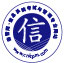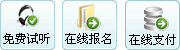﻿ 信息系统项目管理师案例分析每日一练试题（2019/3/14） - 每日一练 - 信管网

[说明][问题1]（8分）

[问题2]（7分）

1答：ev=137000；pv=177000；ac=179600； cv=ev-ac=137000-179600=-42600；cpi=ev/ac=137000/179600=0.76；sv=ev-pv=137000-177000=-40000；spi=ev/pv=137000/177000=0.78； ＜br＞成本超支，进度滞后 ＜br＞ ＜br＞2答：保证成本按照预算的基准内发展，不出现成本超支。成本控制用到的主要工具有：挣值管理，预测，完工尚需绩效指数，储备分析，项目管理系统，输出成本预测，工作绩效信息，变更请求，

1、cv=ev-ac=2000＊100％-2100+5000＊100％-4500+10000＊100％-12000+75000＊90％-86000+65000＊70％-60000+20000＊35％-15000=-44600 ＜br＞   sv=ev-pv=2000＊100％-2000+5000＊100％-5000+10000＊100％-10000+75000＊90％-75000+65000＊70％-65000+20000＊35％-20000=-40000 ＜br＞   cpi=ev/ac=（2000+5000+10000+67500+45000+7500）/（2100+4500+12000+86000+60000+15000）=137000/179600=0.7628 ＜br＞   spi=ev/pv=（2000+5000+10000+67500+45000+7500）/（2000+5000+10000+75000+65000+20000）=137000/177000=0.7740 ＜br＞   成本超出计划，进度偏慢，需要抓紧赶工。 ＜br＞2、成本控制的主要工作内容 ＜br＞   （1）对造成成本基准变更的因素施加影响； ＜br＞   （2）确保所有变更请求都得到及时处理； ＜br＞   （3）当变更实际发生时，管理这些变更； ＜br＞   （4）确保成本支出不超过批准的资金限额，既不超出按时段、按wbs组件、按活动分配的限额，也不超出项目总限额； ＜br＞   （5）监督成本绩效，找出并分析与成本基准间的偏差； ＜br＞   （6）对照资金支出，监督工作绩效； ＜br＞   （7）防止在成本或资源使用报告中出现未经批准的变更； ＜br＞   （8）向有关干系人报告所有经批准的变更及相关成本； ＜br＞   （9）设法把预期的成本超支控制在可接受的范围内。

1、 ＜br＞cv=109500-189600=-80100＜0 成本超支 ＜br＞sv= 109500-165000=-55500＜0 进度滞后 ＜br＞cpi=109500/189600=0.578＜0 成本超支 ＜br＞spi=109500/165000=0.664＜0 进度滞后 ＜br＞2、 ＜br＞需要严格安计划进度执行； ＜br＞控制项目范围； ＜br＞控制项目变更； ＜br＞控制采购成本； ＜br＞ ＜br＞

pv=2000+5000+10000+75000+65000+20000=17700 ＜br＞av=2100+4500+12000+86000+60000+15000=169660 ＜br＞ev=2000＊100％+5000＊100％+10000＊100％+75000＊90％+65000＊70％+20000＊35％=35300 ＜br＞cv=pv-ev=  ＜br＞sv=av-ev  ＜br＞cpi=ev/pv  ＜br＞spi=ev/av

1.cv=179600,ev=137000,pv=133333，sv=ev-pv=36667,cpi=0.76,spi=1.02 ＜br＞成本超支，精度提前 ＜br＞2.制定成本管理计划 ＜br＞  定义活动 ＜br＞  估算成本 ＜br＞  制定预算，建立成本基准 ＜br＞  预算控制 ＜br＞

 发表评论  查看完整评论

### 相关内容8年专注信管，信管教育领跑者，信管网优势免费试听信管网信息系统项目管理师课程全国前50名高分学员访谈：董丽(174)、李思...信息系统项目管理师培训课程(通关班)信管、集成、系规、安全，专业成就梦想信息系统项目管理师历年真题与答案汇总中级系统集成项目管理工程师培训课程系统规划与管理师培训课程（自有资料+直播）软题库：软考在线题库、支持手机答题